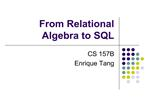DownloadDownload Presentationfrom relational algebra to sql

from relational algebra to sql

Download Presentationfrom relational algebra to sql

- - - - - - - - - - - - - - - - - - - - - - - - - - - E N D - - - - - - - - - - - - - - - - - - - - - - - - - - -
Presentation Transcript

1. From Relational Algebra to SQL CS 157B Enrique Tang

3. Relational Algebra Defined:Tuple An ordered set of data values. { a1 , a2 , a3 , �, an }

4. Relational Algebra Defined: Relation A set of tuples. { { a1, a2, a3, � , an }, { b1, b2, b3, � , bn }, { c1, c2, c3 , � , cn }, ����. ����. }

5. Relational Algebra Defined: Algebra Any formal mathematical system consisting of a set of objects and operations on those objects. Based on operators and a domain of values Operators map arguments from domain into another domain value Example: x = 3.5 * y

6. Relational Algebra Defined: Relational Algebra An algebra whose objects are relations and whose operators transform relations into other relations. Domain: set of relations, i.e., result is another relation Basic operators: select, project, union, set difference, Cartesian product (or cross product)

7. Relational Algebra Defined:Where is it in DBMS?

8. Operations (Unary):Selection, Projection Selection: ? <condition(s)> (<relation>) Picks tuples from the relation Projection: ? <attribute-list> (<relation>) Picks columns from the relation

9. Operations (Set):Union, Set Difference Union: (<relation>) U (<relation>) New relation contains all tuples from both relations, duplicate tuples eliminated. Set Difference: R � S Produces a relation with tuples that are in R but NOT in S.

10. Operations (Set):Cartesian Product, Intersect Cartesian Product: R x S Produces a relation that is concatenation of every tuple of R with every tuple of S The Above operations are the 5 fundamental operations of relational algebra. Intersection: R S All tuples that are in both R and S

11. Operations (Join):Theta Join, Natural Join Theta Join: R F S = ? F (R x S) Select all tuples from the Cartesian product of the two relations, matching condition F When F contains only equality �=�, it is called Equijoin Natural Join: R S Equijoin with common attributes eliminated

12. Operations:Outer Join, Semi Join (left) Outer Join: R S Natural join relations while preserving all tuples from the �outer� side -> NULL values incurred. Semi Join: R F S = ?A (R F S) Join two relations and only keeps the attributes seem in relation R There are Semi-Theta Join, Semi-Equijoin and Semi-Natural Join

13. Operations:Division Division: R � S Produce a relation consist of the set of tuples from R that matches the combination of every tuple in S R S R�S T1 ? ?c (R) T2 ? ?c ((SxT1)�R) T ? T1 � T2

14. Translation to SQL FROM clause produces Cartesian product (x) of listed tables WHERE clause assigns rows to C in sequence and produces table containing only rows satisfying condition ( sort of like ? ) SELECT clause retains listed columns (? )

15. Translation to SQL (Cont.) SELECT C.CrsName FROM Course C, Teaching T WHERE C.CrsCode=T.CrsCode AND T.Sem=�F2003� List CS courses taught in F2003 Tuple variables clarify meaning. Join condition �C.CrsCode=T.CrsCode� eliminates garbage Selection condition � T.Sem=�F2003� � eliminates irrelevant rows Equivalent (using natural join) to: ?CrsName(Course ?Sem=�F2003� (Teaching) ) ?CrsName (?Sem=�F2003� (Course Teaching) )